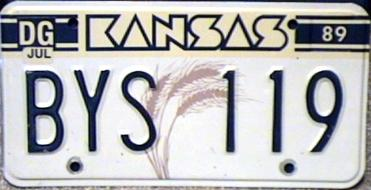# Be careful with your licence plates!

Probability Level 5In Kansas, vehicle licence plates each have exactly three letters followed by three digits. We are told that to produce such a licence plate, it costs $\n$ for each digit $n>0$ and $\10$ for each digit $0$. For letters, the cost is proportional to the position of the letter in the alphabet, namely, $\1$ for $A$, $\2$ for $B$ and so on, up to $\26$ for $Z$.

Determine how many different licence plates would cost exactly $\100$.

×

Problem Loading...

Note Loading...

Set Loading...